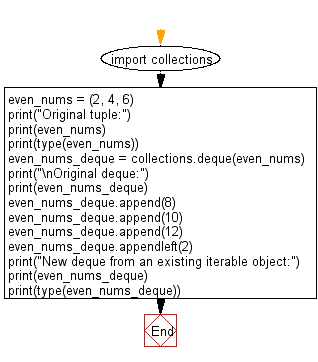﻿ Python: Create a deque from an existing iterable object - w3resource# Python: Create a deque from an existing iterable object

## Python Collections: Exercise-8 with Solution

Write a Python program to create a deque from an existing iterable object.

Sample Solution:

Python Code:

``````import collections
even_nums = (2, 4, 6)
print("Original tuple:")
print(even_nums)
print(type(even_nums))
even_nums_deque = collections.deque(even_nums)
print("\nOriginal deque:")
print(even_nums_deque)
even_nums_deque.append(8)
even_nums_deque.append(10)
even_nums_deque.append(12)
even_nums_deque.appendleft(2)
print("New deque from an existing iterable object:")
print(even_nums_deque)
print(type(even_nums_deque))
```
```

Sample Output:

```Original tuple:
(2, 4, 6)
<class 'tuple'>

Original deque:
deque([2, 4, 6])
New deque from an existing iterable object:
deque([2, 2, 4, 6, 8, 10, 12])
<class 'collections.deque'>
```

Flowchart:## Visualize Python code execution:

The following tool visualize what the computer is doing step-by-step as it executes the said program:

Python Code Editor:

Have another way to solve this solution? Contribute your code (and comments) through Disqus.

What is the difficulty level of this exercise?

Test your Programming skills with w3resource's quiz.

﻿

## Python: Tips of the Day

Use Reversed() In for Loops:

```>>> tasks = ['laundry', 'picking up kids', 'gardening', 'cooking']